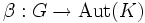# Action-isomorph-free subgroup

## Contents

BEWARE! This term is nonstandard and is being used locally within the wiki. [SHOW MORE]
This article defines a subgroup property: a property that can be evaluated to true/false given a group and a subgroup thereof, invariant under subgroup equivalence. View a complete list of subgroup properties[SHOW MORE]

## Definition

### Definition with symbols

A subgroup$H$ of a group$G$ is termed action-isomorph-free in$G$ if$H$ is a normal subgroup of$G$, and the following condition holds.

Suppose$\alpha:G \to \operatorname{Aut}(H)$ be the homomorphism induced by the action of$G$ on$H$ by conjugation. Suppose$K$ is a normal subgroup of$G$ with$\beta:G \to \operatorname{Aut}(K)$ the homomorphism induced by the action of$G$ on$K$ by conjugation. Suppose, further, that$\sigma:H \to K$ is an isomorphism with the property that: